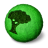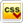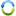TERMSOOK.COM Slogan slogan slogan
 Simple simple simple simple simple simple simple simple simple simple simple simple simple simple simple simple simple simple simple simple simple simple simple simple simple simple simple simple simple simple simple simple simple simple simple simple simple simple simple simple simple simple simple simple simple simple simple simple simple simple simple simple simple simple simple simple simple simple simle
***Note: Right click here. And selectfor Edit header image ***SearchMain MenuCounterสถิติวันนี้ 33 คนสถิติเมื่อวาน 19 คนสถิติเดือนนี้สถิติปีนี้สถิติทั้งหมด 579 คน17656 คน585030 คน เริ่มเมื่อ 2009-11-26Link